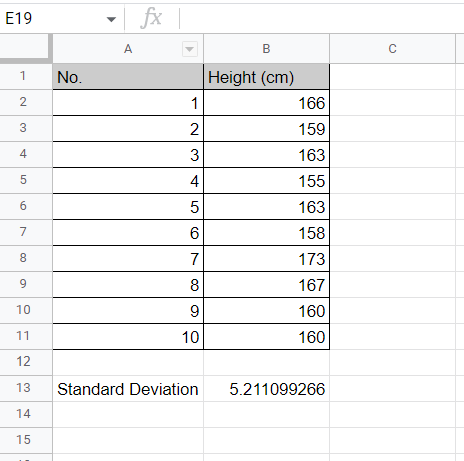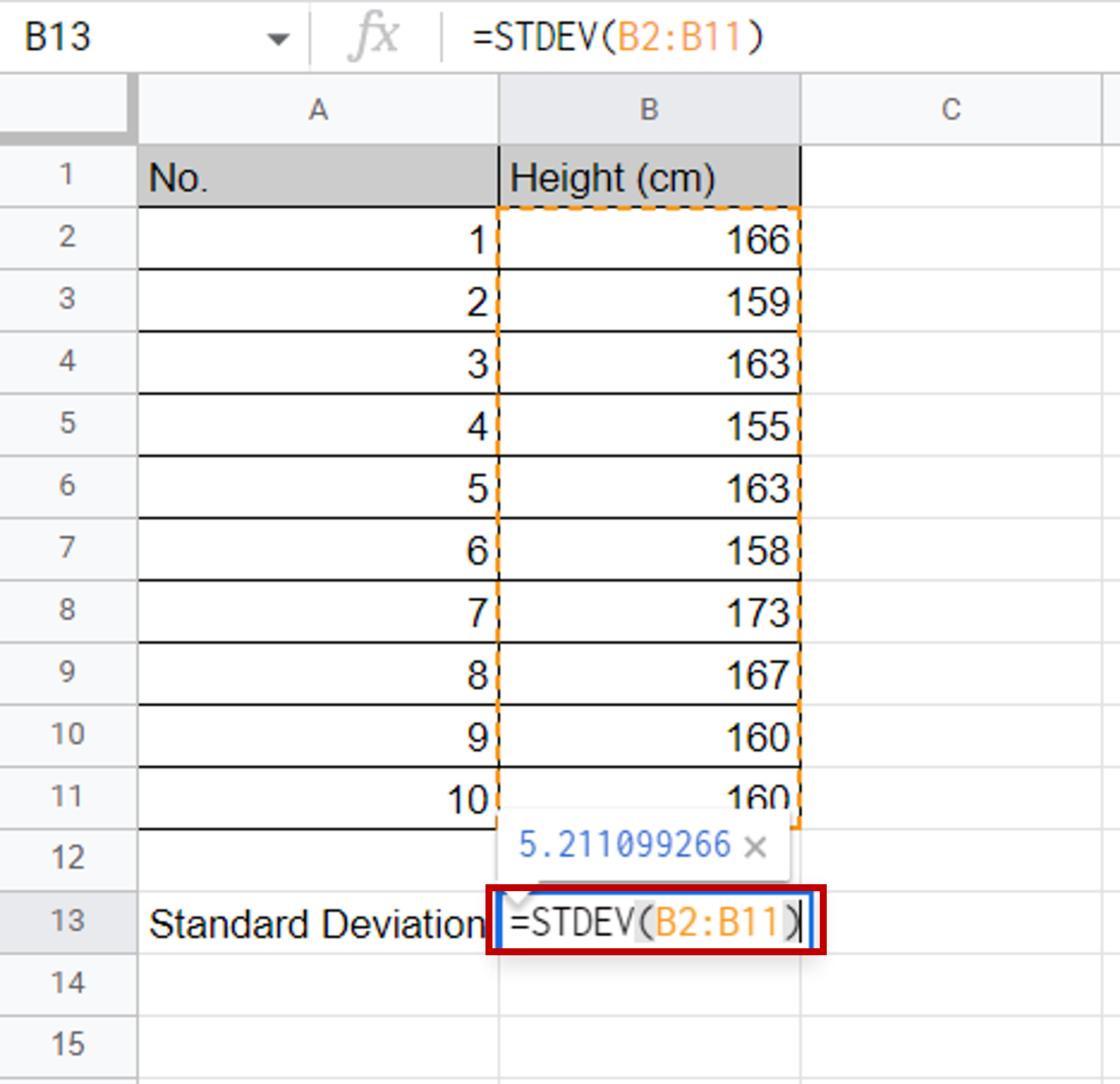# How to find standard deviation in Google sheets

You can watch a video tutorial here.The standard deviation of a set of values is an invaluable metric for understanding how the data is distributed. The standard deviation measures how far the values in a dataset are from the mean or average and so tells you how spread out the data is. If the number is high, then the data is widely spread out but if it is low, then the data is distributed closer to the mean. Although you can calculate the standard deviation using the mathematical formula in Google sheets, it is easier to do this using the function provided.

### Step 1 – Type the formula– In the destination cell, type the formula:
=stdev(<range of values>)

### Step 2 – Check the result– Check the value of the standard deviation to understand how spread out the data is